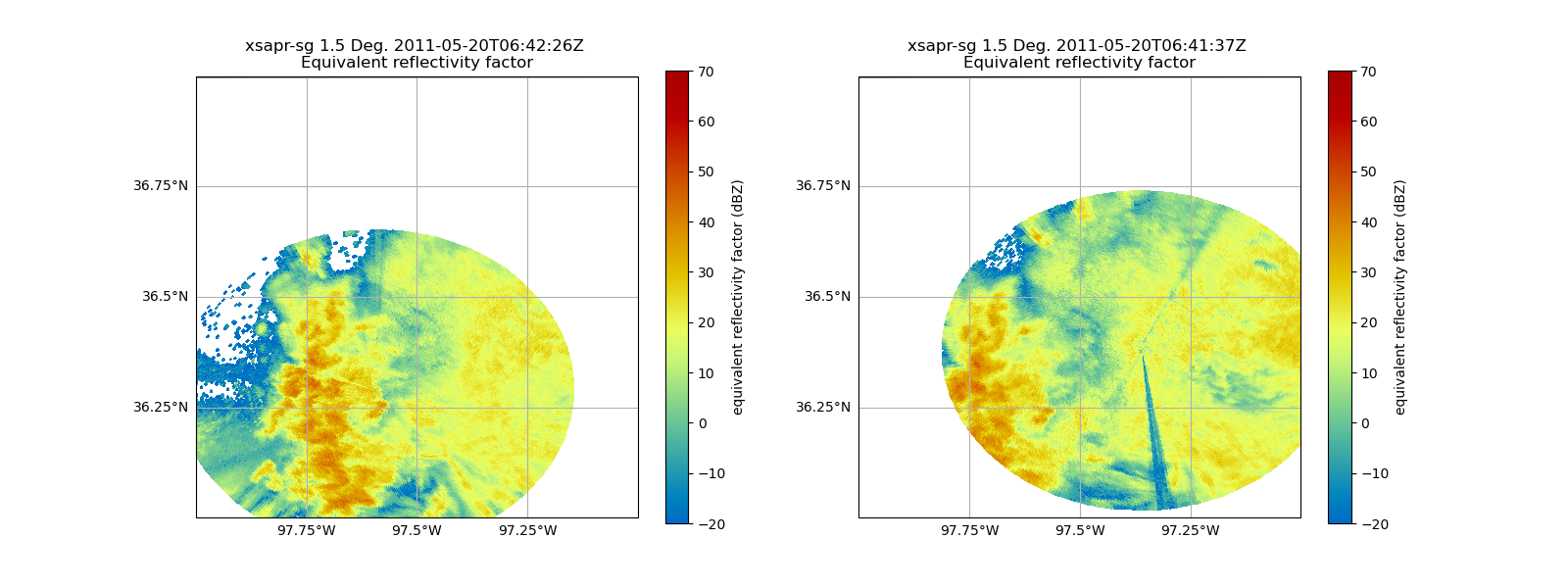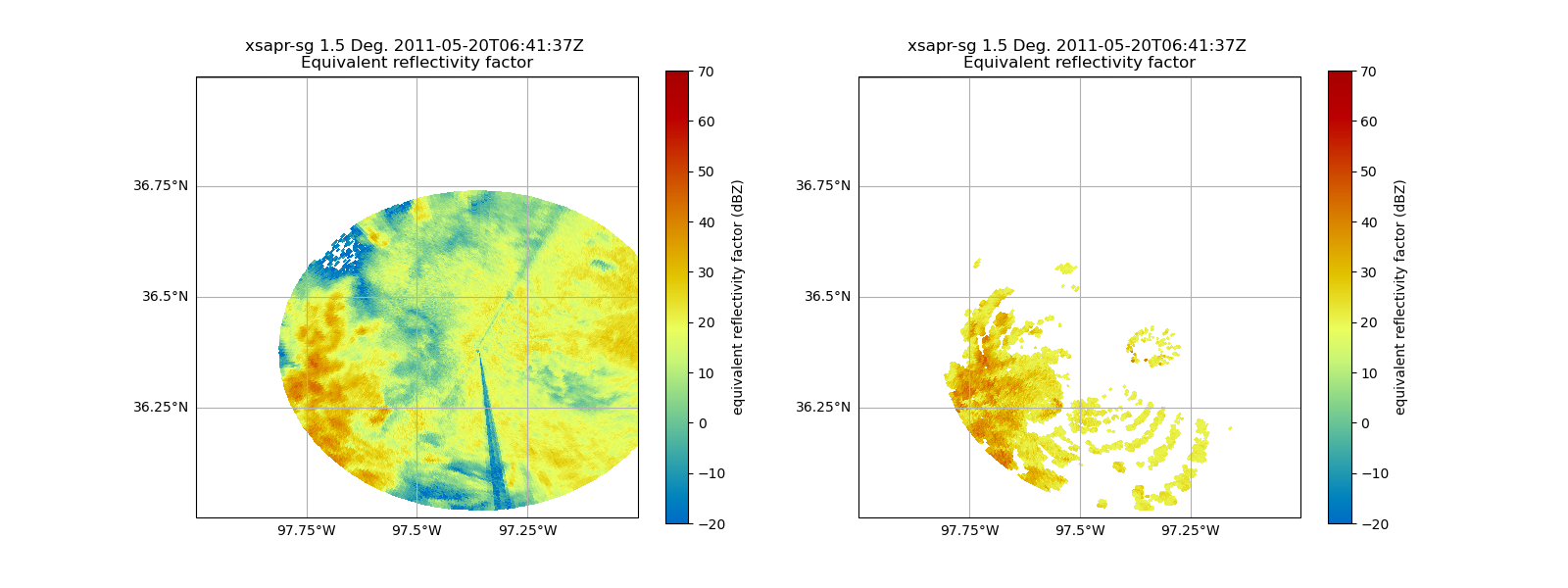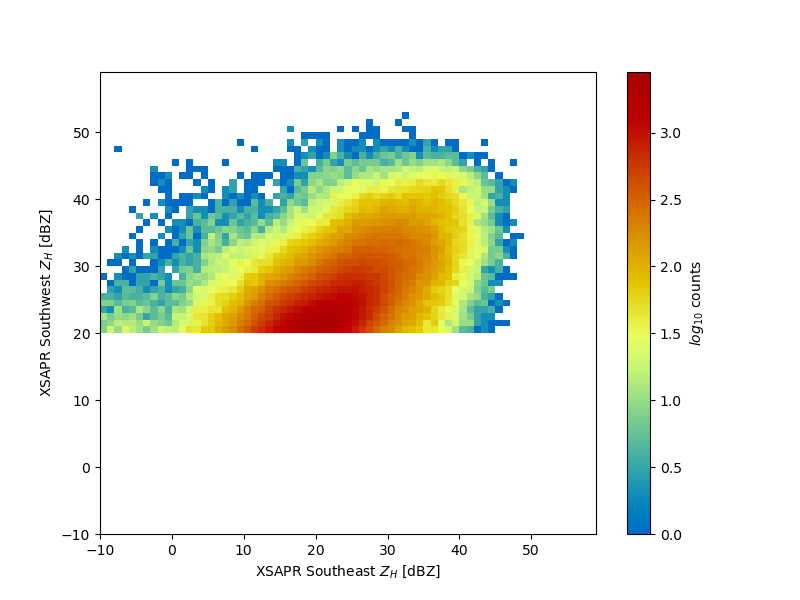# Compare Two Radars Using Gatemapper#

Map the reflectivity field of a single radar in Antenna coordinates to another radar in Antenna coordinates and compare the fields.

```print(__doc__)

# Author: Max Grover (mgrover@anl.gov) and Bobby Jackson (rjackson@anl.gov)

import warnings

import cartopy.crs as ccrs
import matplotlib.pyplot as plt
import numpy as np

import pyart
from pyart.testing import get_test_data

warnings.filterwarnings("ignore")
```

For this example, we use two XSAPR radars from our test data.

```# read in the data from both XSAPR radars
xsapr_sw_file = get_test_data("swx_20120520_0641.nc")
xsapr_se_file = get_test_data("sex_20120520_0641.nc")
```

Filter and Configure the GateMapper

We are interested in mapping the southwestern radar to the southeastern radar. Before running our gatemapper, we add a filter for only positive reflectivity values. We also need to set a distance (meters) and time (seconds) between the source and destination gate allowed for an adequate match), using the distance_tolerance/time_tolerance variables.

```gatefilter = pyart.filters.GateFilter(radar_sw)
gatefilter.exclude_below("reflectivity_horizontal", 20)
gmapper = pyart.map.GateMapper(
distance_tolerance=500.0,
time_tolerance=60,
gatefilter_src=gatefilter,
)
```

Plot the Original Data

Let’s take a look at our original fields - notice the difference in reflectivity values!

```fig = plt.figure(figsize=(16, 6))
ax = plt.subplot(121, projection=ccrs.PlateCarree())

disp1.plot_ppi_map(
"reflectivity_horizontal",
sweep=1,
ax=ax,
vmin=-20,
vmax=70,
min_lat=36,
max_lat=37,
min_lon=-98,
max_lon=-97,
lat_lines=np.arange(36, 37.25, 0.25),
lon_lines=np.arange(-98, -96.75, 0.25),
)

ax2 = plt.subplot(122, projection=ccrs.PlateCarree())
disp2.plot_ppi_map(
"reflectivity_horizontal",
sweep=1,
ax=ax2,
vmin=-20,
vmax=70,
min_lat=36,
max_lat=37,
min_lon=-98,
max_lon=-97,
lat_lines=np.arange(36, 37.25, 0.25),
lon_lines=np.arange(-98, -96.75, 0.25),
)
```Now, we can compare our original field from the southwestern radar, to the new remapped field - there are similarities…

```fig = plt.figure(figsize=(16, 6))
ax = plt.subplot(121, projection=ccrs.PlateCarree())

disp1.plot_ppi_map(
"reflectivity_horizontal",
sweep=1,
ax=ax,
vmin=-20,
vmax=70,
min_lat=36,
max_lat=37,
min_lon=-98,
max_lon=-97,
lat_lines=np.arange(36, 37.25, 0.25),
lon_lines=np.arange(-98, -96.75, 0.25),
)

ax2 = plt.subplot(122, projection=ccrs.PlateCarree())
disp2.plot_ppi_map(
"reflectivity_horizontal",
sweep=1,
ax=ax2,
vmin=-20,
vmax=70,
min_lat=36,
max_lat=37,
min_lon=-98,
max_lon=-97,
lat_lines=np.arange(36, 37.25, 0.25),
lon_lines=np.arange(-98, -96.75, 0.25),
)
```Calculate and Plot the Difference

It can be difficult to “eyeball” the difference between these two fields. Fortunately, now that our radars match coordinates, we can plot a difference. Keep in mind there is a time difference of ~ 1 minute between these plots, leading to small difference due to the precipitation moving through the domain over the course of that minute.

```# Extract the numpy arrays for our reflectivity fields
"data"
]

# Calculate the difference between the southeastern and southwestern radar

"reflectivity_horizontal",
field_name="reflectivity_bias",
data=reflectivity_difference,
)

# Setup our figure
fig = plt.figure(figsize=(8, 6))
ax = plt.subplot(111, projection=ccrs.PlateCarree())

# Plot the difference field
disp1.plot_ppi_map(
"reflectivity_bias",
cmap="pyart_balance",
title="Reflectivity Difference \n XSAPR Southwest - XSPAR Southeast",
sweep=1,
ax=ax,
vmin=-30,
vmax=30,
min_lat=36,
max_lat=37,
min_lon=-98,
max_lon=-97,
lat_lines=np.arange(36, 37.25, 0.25),
lon_lines=np.arange(-98, -96.75, 0.25),
)
```Plot a Histogram for Comparison

Another way of plotting the comparison here is using a 2-dimensional histogram,which is more helpful in this case where our scans don’t neccessarily match exactly in time.

```# Include elevations above the lowest one

# Filter the reflectivity fields using the filter created above

# Make sure not include masked values

# Set the bins for our histogram
bins = np.arange(-10, 60, 1)

# Create the 2D histogram using the flattened numpy arrays
hist = np.histogram2d(refl_se.flatten(), refl_sw.flatten(), bins=bins)
hist = np.ma.masked_where(hist == 0, hist)

# Setup our figure
fig = plt.figure(figsize=(8, 6))

# Create a 1-1 comparison
x, y = np.meshgrid((bins[:-1] + bins[1:]) / 2.0, (bins[:-1] + bins[1:]) / 2.0)
c = plt.pcolormesh(x, y, np.log10(hist.T), cmap="pyart_HomeyerRainbow")

# Add a colorbar and labels
plt.colorbar(c, label="\$log_{10}\$ counts")
plt.xlabel("XSAPR Southeast \$Z_{H}\$ [dBZ]")
plt.ylabel("XSAPR Southwest \$Z_{H}\$ [dBZ]")

plt.show()
```Total running time of the script: (0 minutes 50.545 seconds)

Gallery generated by Sphinx-Gallery